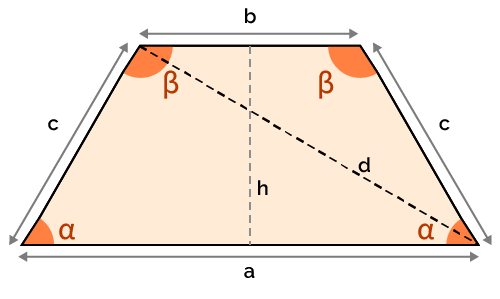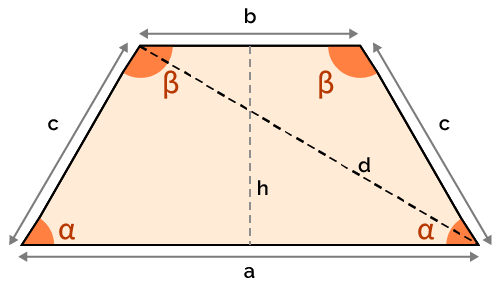# Isosceles Trapezoid Calculator

Created by Anna Szczepanek, PhD
Reviewed by Davide Borchia
Last updated: Sep 30, 2022

Welcome to Omni's isosceles trapezoid calculator! Here you can learn what an isosceles trapezoid is and explore the various properties of such trapezoids. In particular, we'll explain how to compute the height and diagonal of an isosceles trapezoid.

Let us kick off with the definition of an isosceles trapezoid.

## What is an isosceles trapezoid?

An isosceles trapezoid is a trapezoid with legs that have the same length (compare to isosceles triangles).

Just in case, let us also recall that a trapezoid is a geometric shape with four sides such that at least one pair of sides is parallel to each other. If there are two such pairs, then we get a parallelogram. The two parallel sides are called bases, and the other two sides are called legs.That's it when it comes to the definition of isosceles trapezoids! Let's explore some interesting properties of these intriguing geometric objects.

## What are the properties of isosceles trapezoids?

Here's a brief overview of the main properties of an isosceles trapezoid:

• The diagonals of an isosceles trapezoid are of equal length.
• But these diagonals do not necessarily bisect each other.
• The base angles are the same.
• An isosceles trapezoid which is also a right trapezoid is a rectangle.
• An isosceles trapezoid has an axis of symmetry - the line of symmetry goes through the midpoints of the bases.
• But an isosceles trapezoid has no rotational symmetry (unless it is a rectangle).
• The sum of opposite angles is equal to the straight angle (180 degrees).

What is the best way to explore all these different properties? Experimenting with our isosceles trapezoid calculator! In the next section, we explain how to use it most effectively.

## How to use this isosceles trapezoid calculator?

Nothing can be easier than using Omni's isosceles trapezoid calculator! You just need to input the data that is available (in any order), and our tool will find all the other values.

Mind that the calc operates under the assumption that the longer basis is denoted by a, and the shorter basis by b (they may of course be equal)!

## Useful trapezoid resources

Omni has lots of other calculators that will solve your trapezoid problems:

## FAQ

### Is an isosceles trapezoid a parallelogram?

An isosceles trapezoid need not be a parallelogram. We need to additionally know that the two bases of the isosceles trapezoid in question are of the same length.

### How many lines of symmetry does this isosceles trapezoid have?

An isosceles trapezoid has exactly one line of symmetry. It can be found by drawing a line through the midpoints of the two bases of our isosceles trapezoid.

Anna Szczepanek, PhDLonger base (a)
in
Shorter base (b)
in
Leg (c)
in
Height (h)
in
Acute angle (α)
deg
Obtuse angle (β)
deg
Diagonal (d)
in
Perimeter
in
Area
in²
People also viewed…

### Black Friday

How to get best deals on Black Friday? The struggle is real, let us help you with this Black Friday calculator!

### Cross product

Cross product calculator finds the cross product of two vectors in a three-dimensional space.

### Long division

Check this long division calculator if you want to solve any division problem and see all the steps towards the result.

### Social Media Time Alternatives

Check what you could have accomplished if you get out of your social media bubble.